# 1. Generate table model for single table operation (models.py)

Complete `MySQL`the configuration of other databases first , and then perform other operations on the data table, so that the data can be saved to `MySQL`, otherwise it will be saved to the default database`SQLite`

`models.py`Create a table model in the current application

``````from django.db import models
class Book1(models.Model):
id = models.AutoField(primary_key=True) # AutoField自动增加
book_name = models.CharField(max_length=32)
pub_date = models.DateField()
# 浮点数，8位数，其中有两位是小数学
price = models.DecimalField(max_digits=8,decimal_places=2)
publish = models.CharField(max_length=32)
``````
• Execute the data migration command to generate this table in the database
``````# 在Pycharm中的Terminal中输入命令
py manage.py makemigrations
py manage.py migrate
``````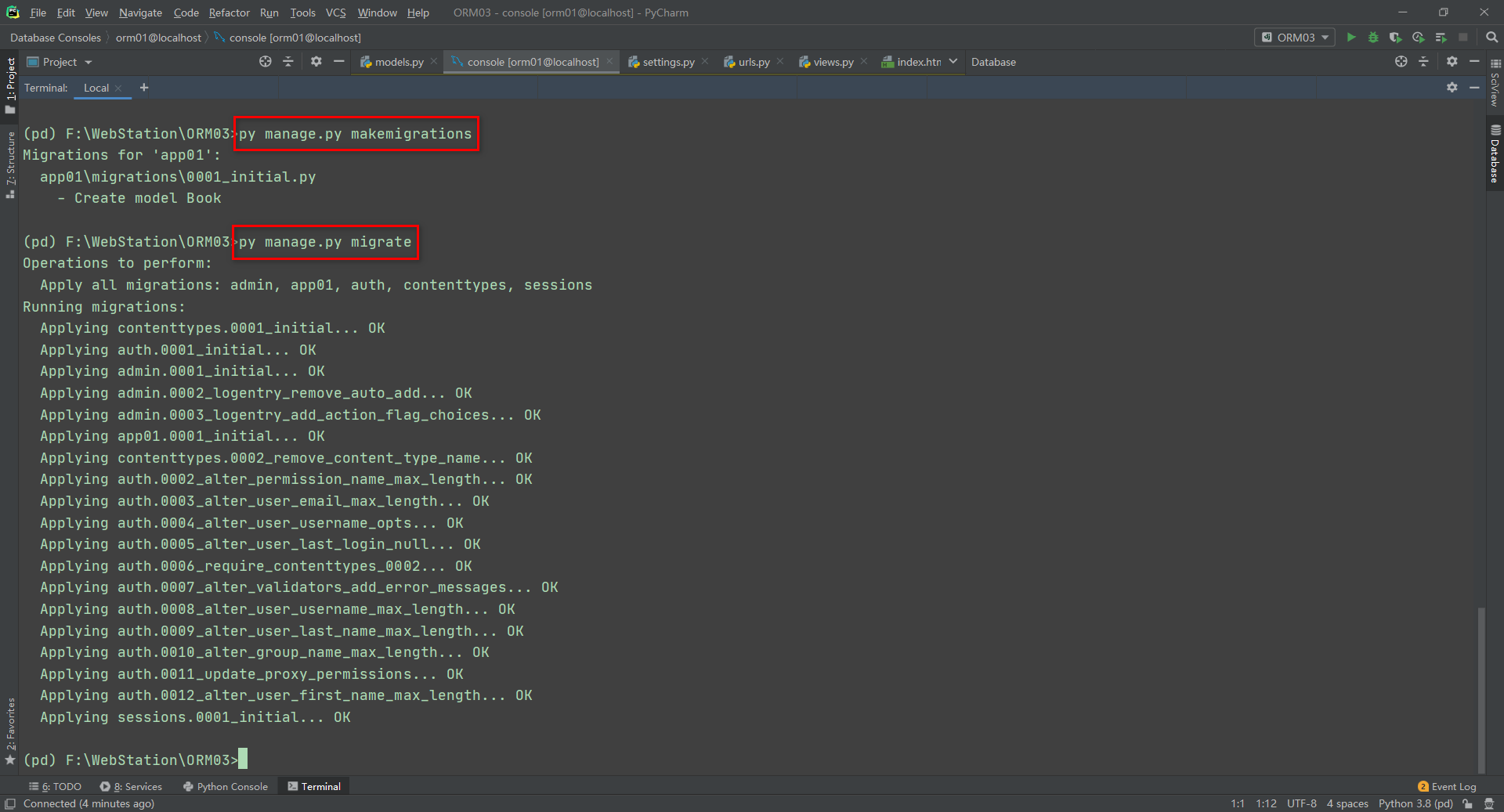• After `Pycharm`logging in in `MySQL`China, query through the following methods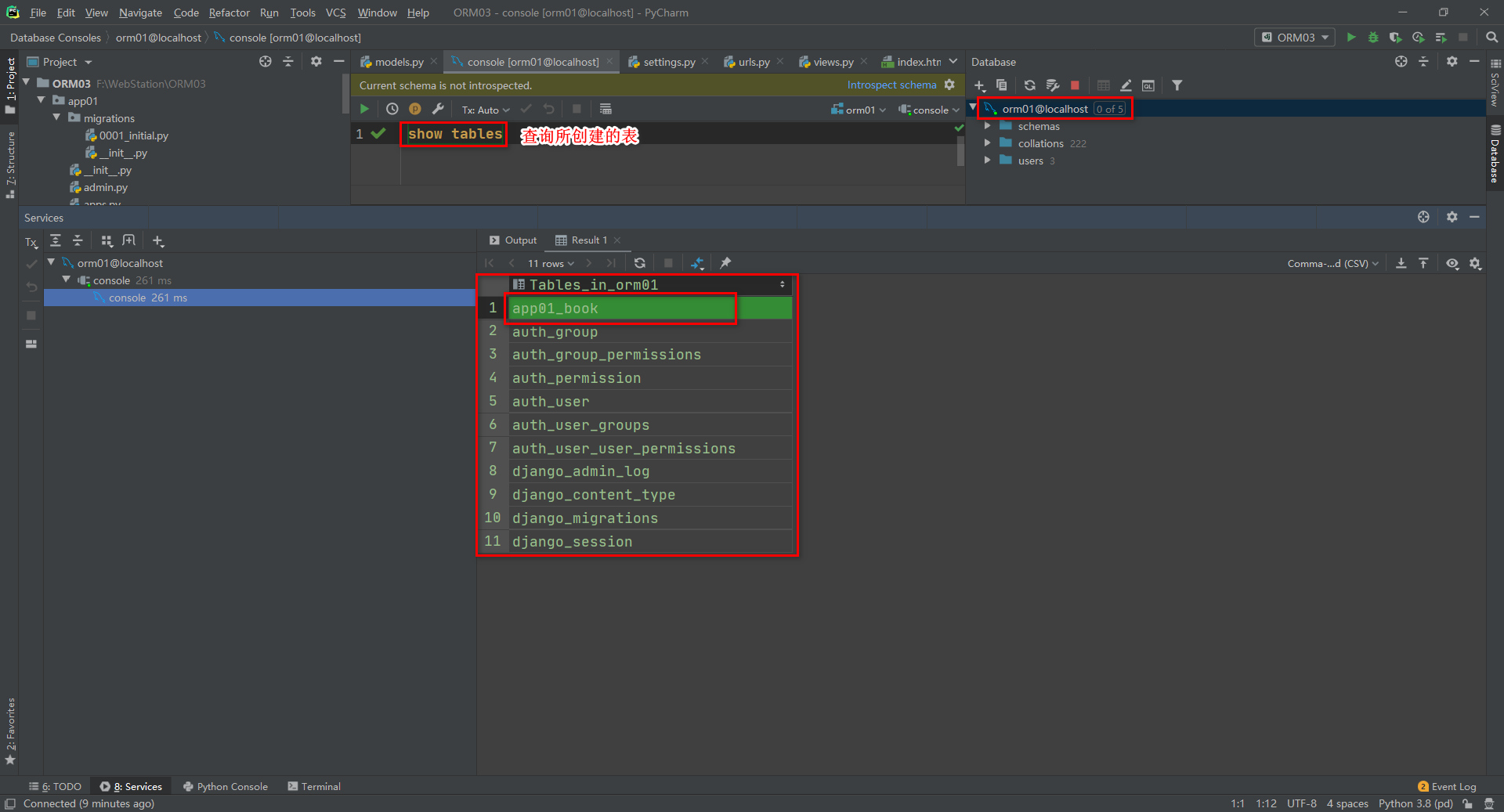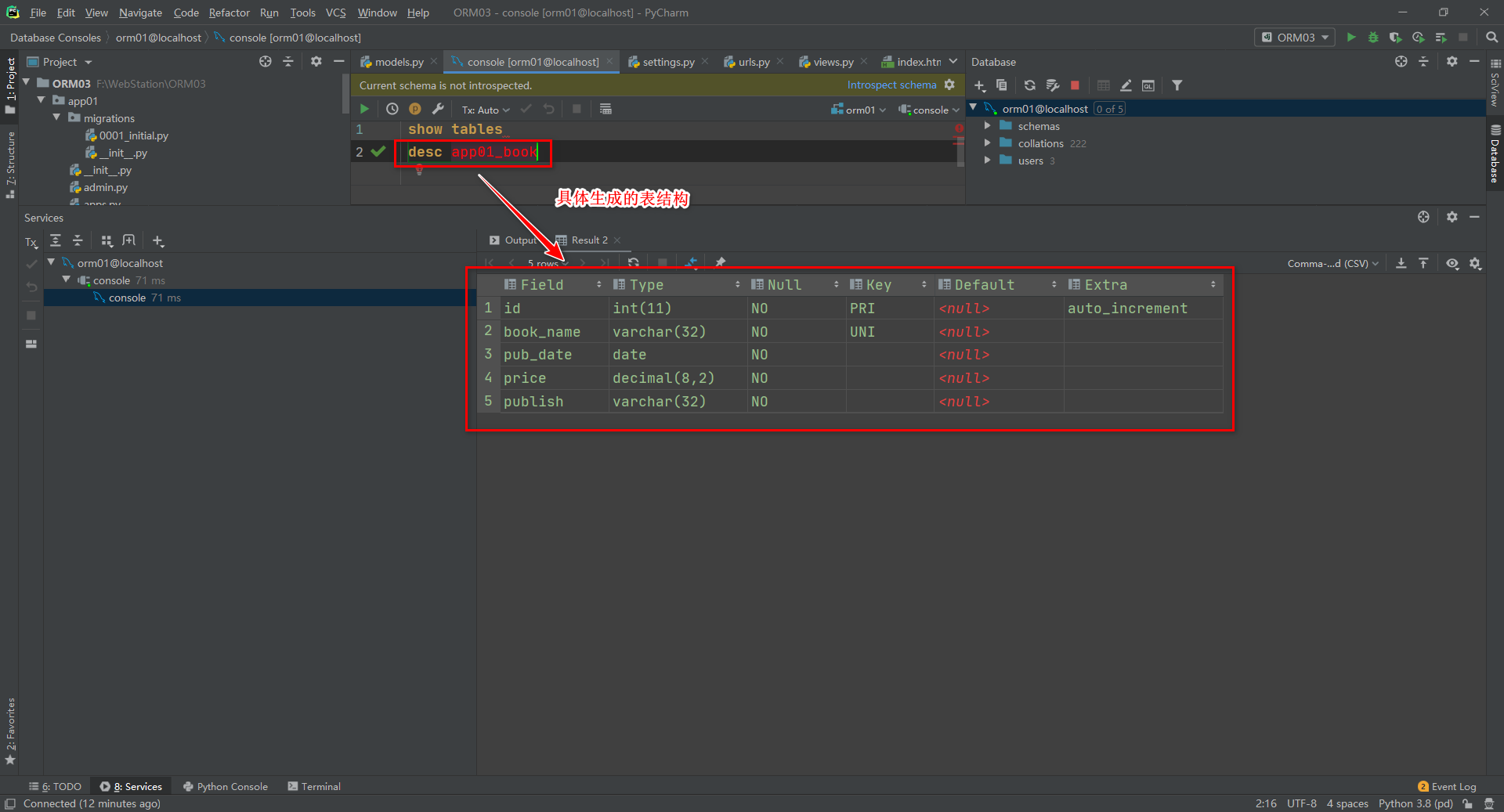# 2. Add record for single table operation (views.py)

• method one:
``````from app01.models import Book
from Django.shortcuts import render,HttpRespose
def index(request):
book_obj = Book(id=1,book_name="python",price=99.8,
pub_date="2012-12-06",publish="重庆出版社")
book_obj.save()
return HttpRespose("保存成功")
``````
• Way two:
``````from app01.models import Book
from Django.shortcuts import render,HttpRespose
def index(request):
book_obj = Book.object.create(id=1,book_name="python",price=99.8,pub_date="2012-12-06",publish="重庆出版社")
return HttpRespose("保存成功")
``````
• `Pycharm`In the connection `MySQL`, view the added records as follows: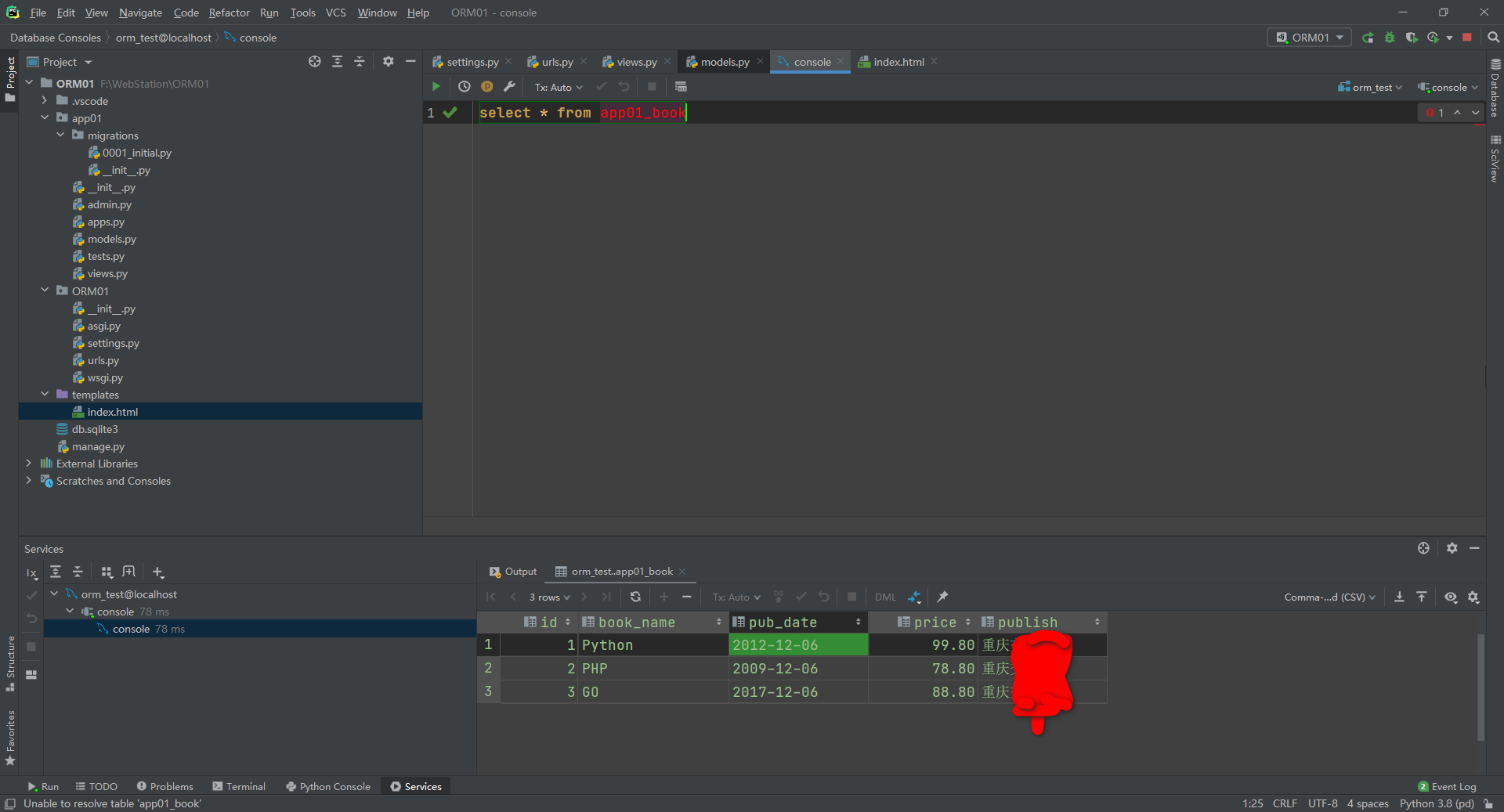• `MySQL`View tools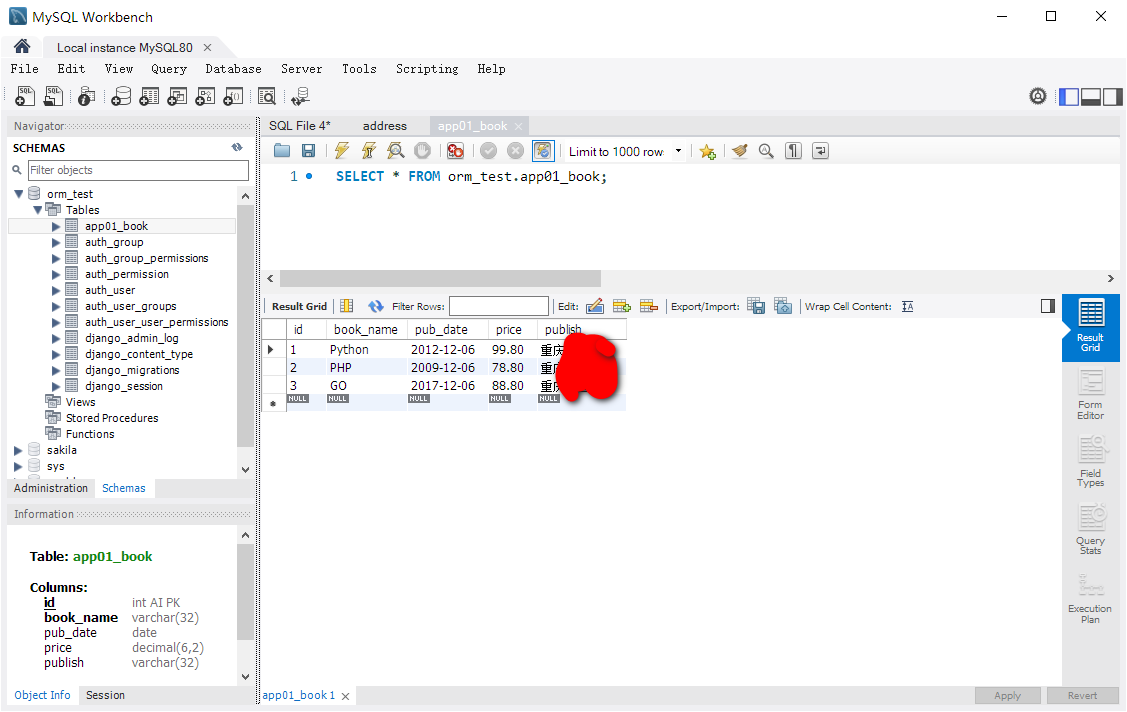# 3. Query record of single table operation (views.py)

## 3.1 all() returns all objects in the table

• The caller is `object`(manager object), `all()`the return value of the method is an `queryset`object, and the `queryset`data structure is similar to a list, containing all objects in the table
``````"""*****查询表记录*****"""
book_list = Book.objects.all()
for i in book_list:
print(i,"\t",i.pub_date)
print("输出完毕")
print(book_list.publish)
``````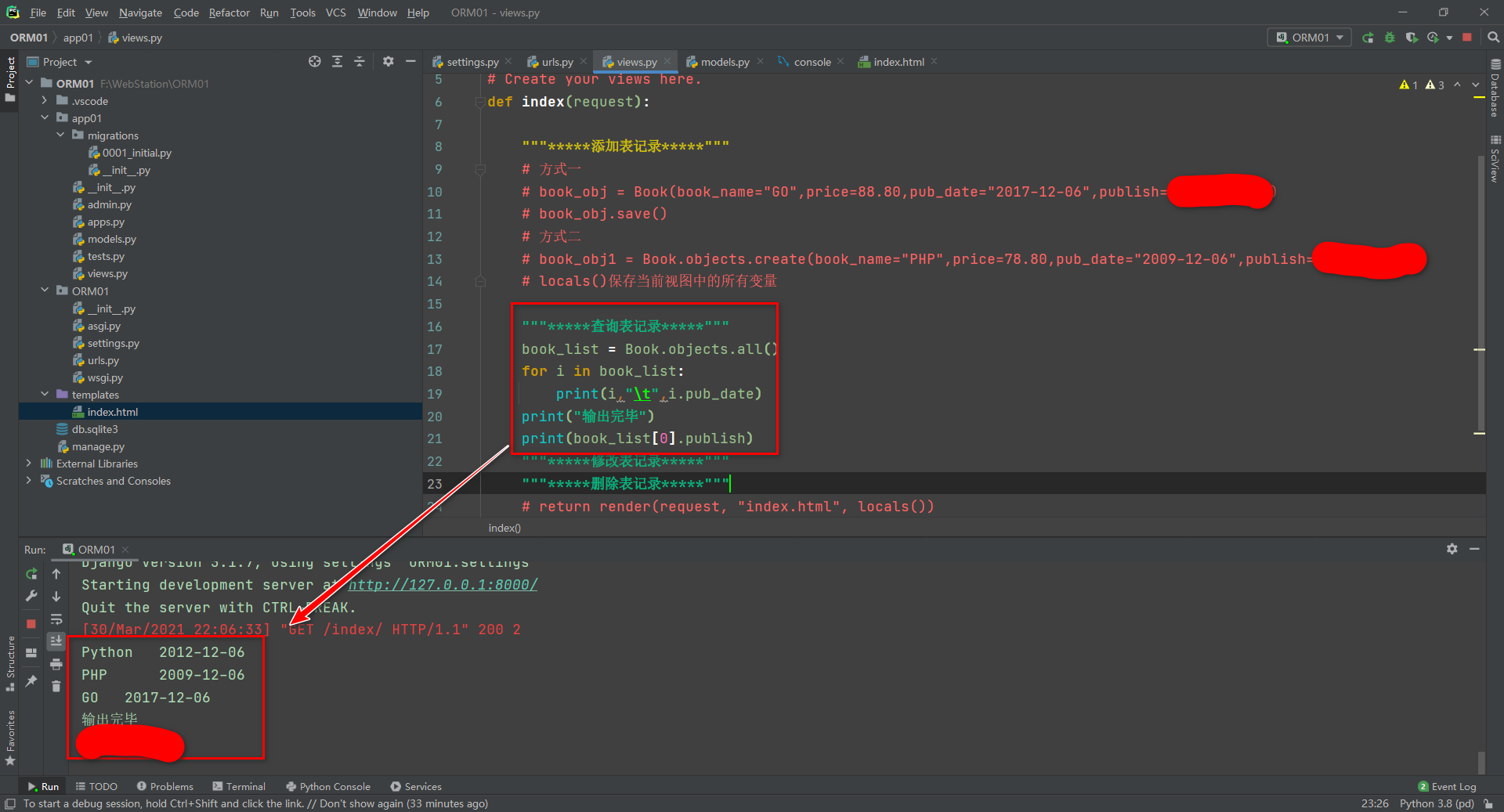## 3.2 first() and last() return the first and last results of the object, respectively

The caller is an `queryset`object, and the return value is an `model`object

Note: you cannot use that `book.objects`(manager object) to call

``````"""*****first()*****"""
book = Book.objects.all().first()
# 等同于
book = Book.objects.all()

"""*****last()*****"""
book = Book.objects.all().last()
# 等同于
book = Book.objects.all()[-1]
``````

## 3.3 filter() returns the filtered result

• The caller can be an `queryset`object or directly `object`(manager object), and the return value is an `queryset`object
``````book_list = Book.objects.filter(book_name="Python")
book_list = Book.objects.all().filter(book_name="Python")
``````

## 3.4 get() only one result is a model object

• Caller: it can be an `queryset`object or `object`(manager object), return value: only one result is meaningful, the result is an `model`object
``````book_obj = Book.objects.all().get(book_name="Python",publish="重庆交通大学")
print(book_obj.pub_date)
``````

## 3.5 exclude() returns the opposite result of the filter() method

• Caller: it can be an `queryset`object or `object`(manager object), return value: both are an `queryset`object
• All results except what
``````book_list = Book.objects.exclude(book_name="Python")
book_list = Book.objects.all().exclude(book_name="Python")
``````

## 3.6 order_by() returns the sorted result

• Caller: it can be an `queryset`object or `object`(manager object), return value: both are an `queryset`object
``````book_list = Book.objects.all().order_by("price") # 升序
book_list1 = Book.objects.order_by("-price",'-id') # 降序
print(book_list)
print(book_list1)
``````

## 3.7 reverse() returns the result in reverse order

Caller: it can be an `queryset`object or `object`(manager object), return value: both are an `queryset`object

With `order_by`opposite results sorted

``````book_list = Book.objects.reverse().order_by("-price")
book_list1 = book_list.reverse()
print(book_list)
print(book_list1)
``````

## 3.8 count() returns an int value that meets the requirements

• Caller: it can be a `queryse`t object or `object`(manager object), return value: both are a `int`number
``````ret = Book.objects.count()
print(ret)
``````

## 3.9 exist() returns True if it exists

• Caller: it can be an `queryset`object or `object`(manager object), return value: a `bool`value
``````ret = Book.objects.exist()
print(ret)
``````

## 3.10 values() only returns the value corresponding to the field (dictionary)

• Caller: it can be an `queryset`object or `object`(manager object), return value: both are an `queryset`object
``````# 引例
# ret = Book.objects.all()
# print([i.book_name for i in ret])
ret = Book.objects.all().values("price","publish")
print(ret)
``````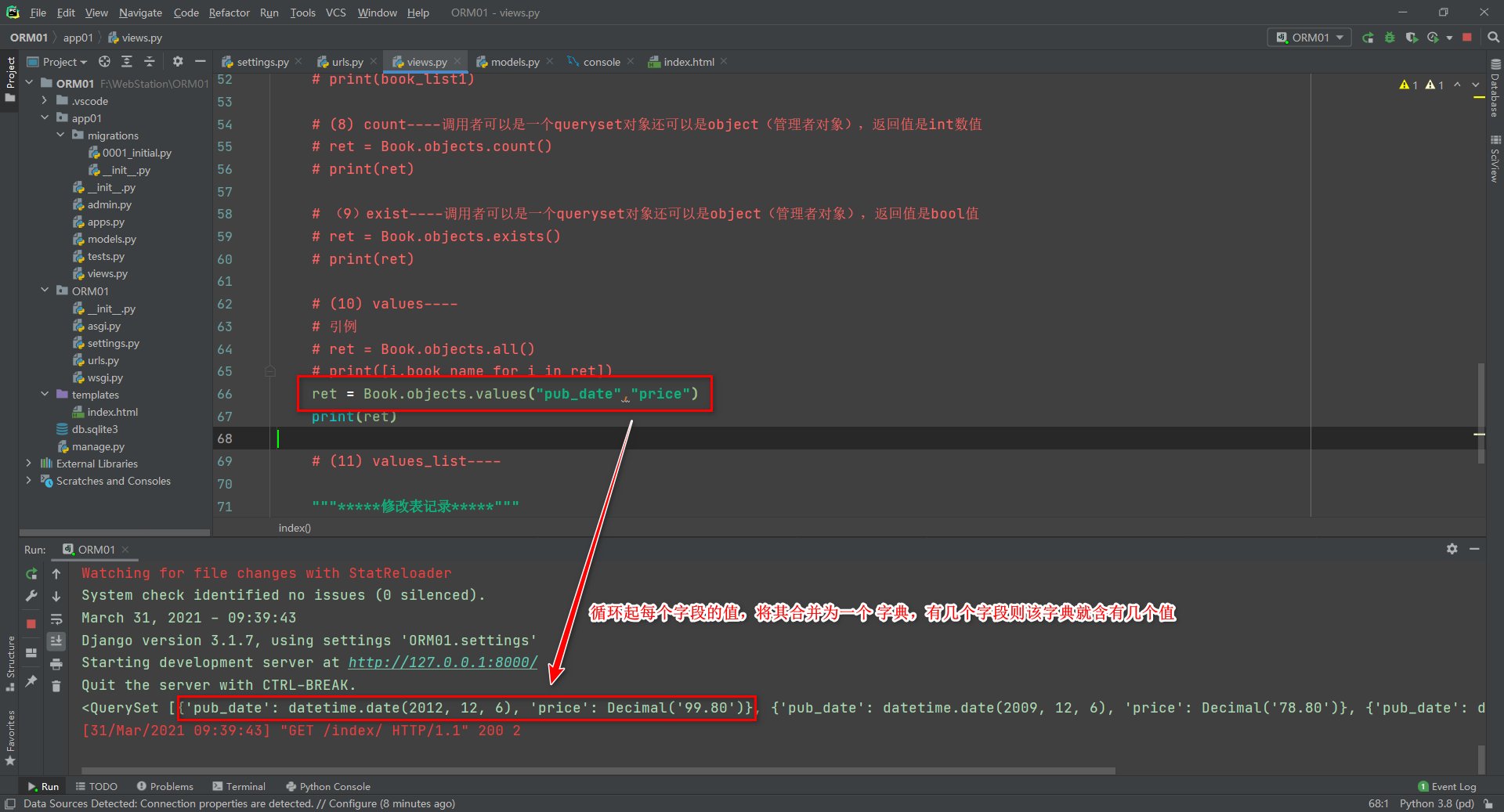• Cycle through the values ​​of each field and merge them into a dictionary. If there are several fields, the dictionary contains several values
• The premise is to include this field, and place the ones belonging to the peer under the same dictionary

## 3.11 values_list() only returns the value (tuple) corresponding to the field

• The caller can be an `queryset`object or `object`(manager object), and the return value is an `queryset`object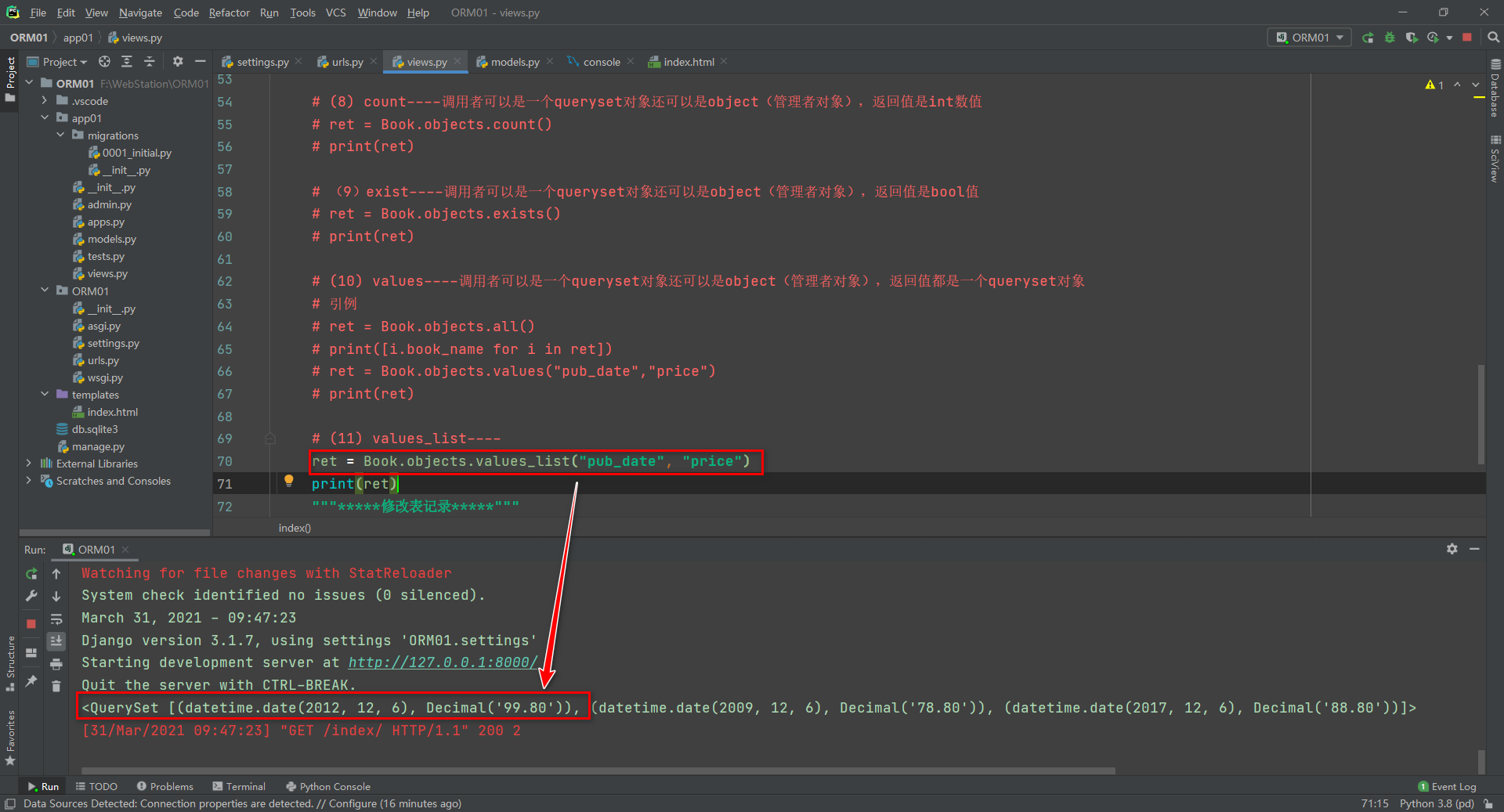• The `values()`difference is: `values`inside is a dictionary, `values_list`inside is a tuple

## 3.12 distinct() de-duplication, only makes sense for one field

• Caller: it can be an `queryset`object or `object`(manager object), return value: both are an `queryset`object
``````ret = Book.objects.values("price").distinct()
ret1 = Book.objects.values("price")
print(ret)
print(ret1)
``````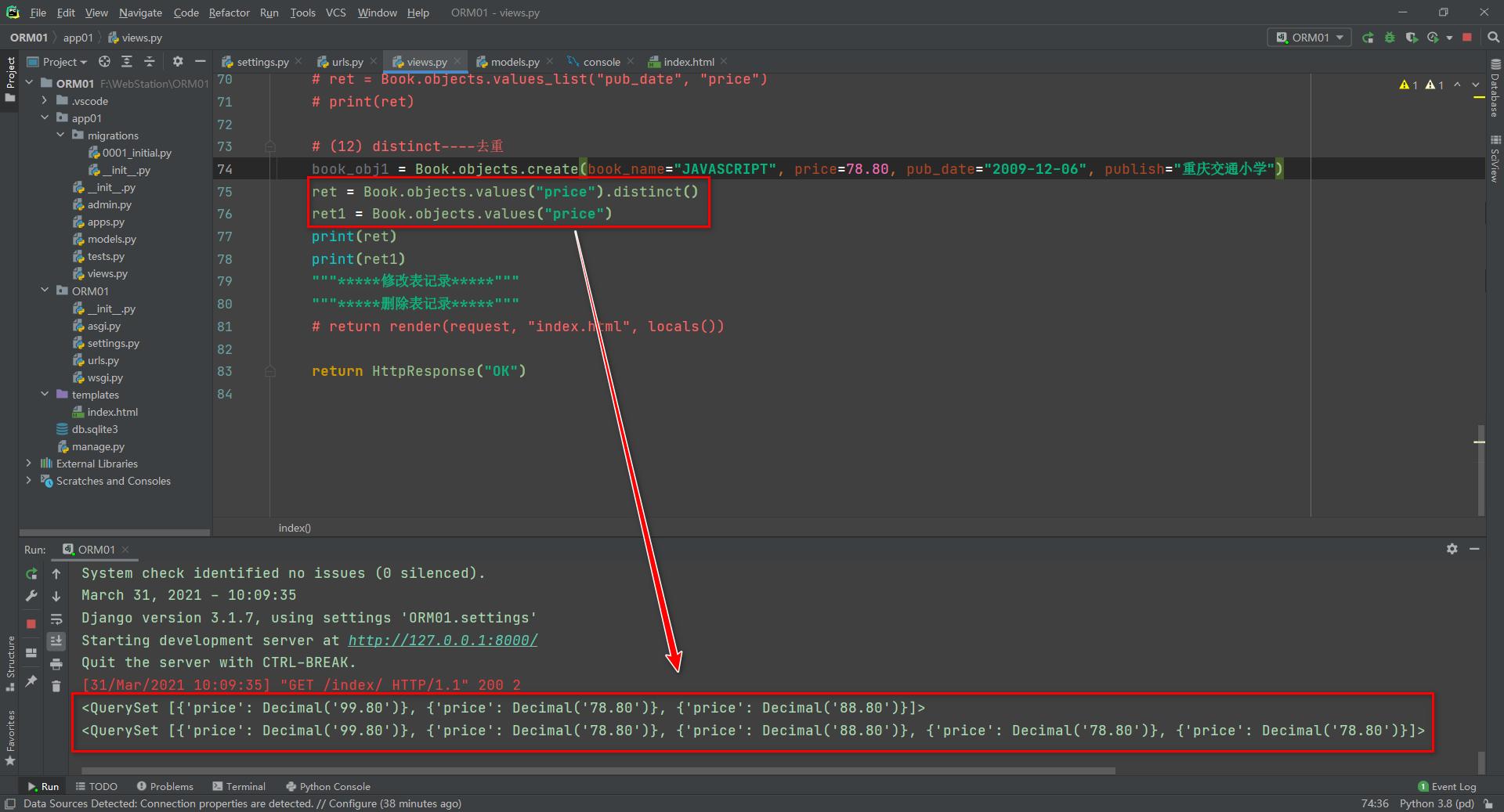• Need to cooperate `values`or `values_list`use
• Multiple fields can also be used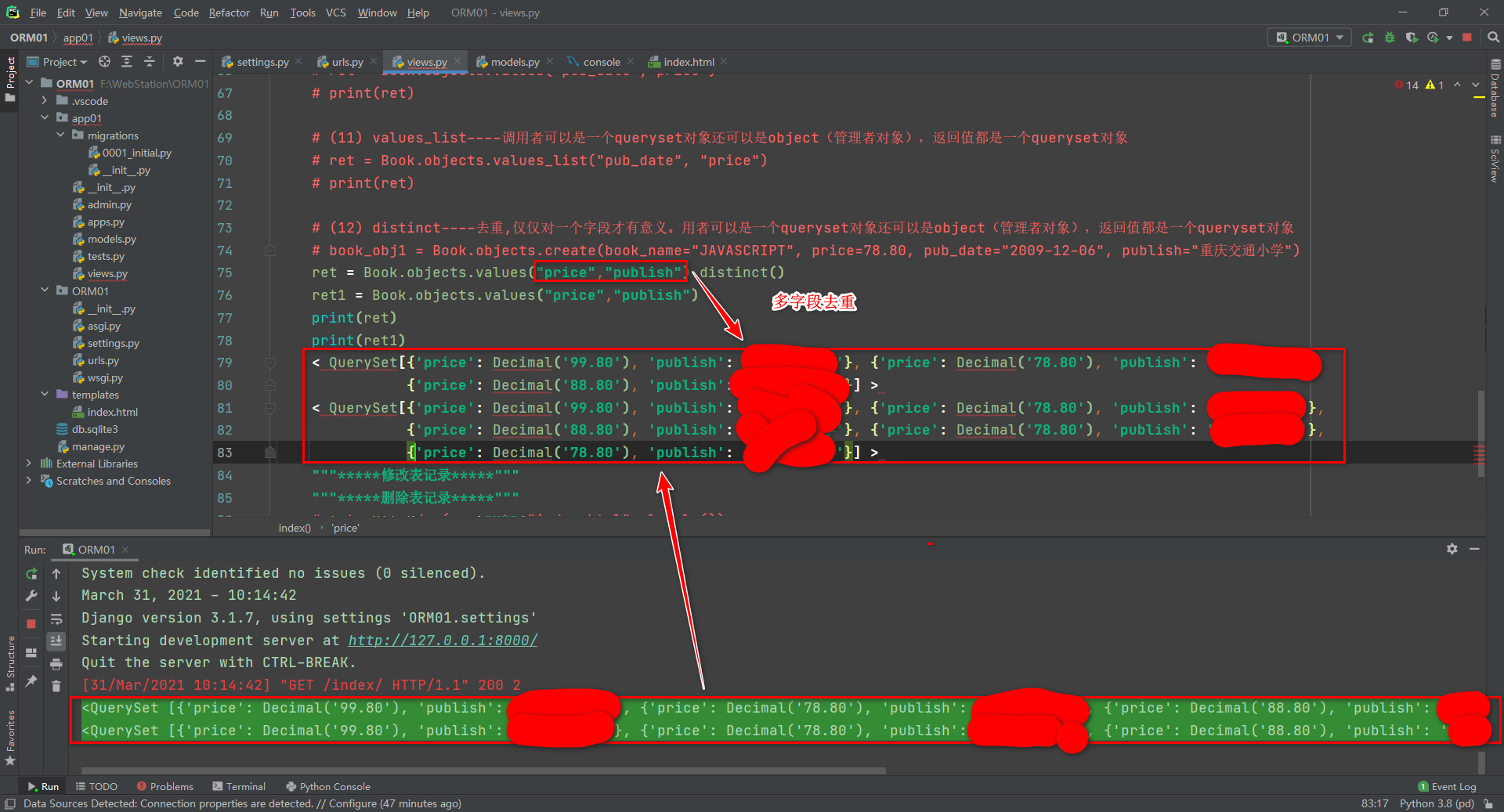# 4. Filter() fuzzy query for single table query

• `filter()`Method is based on fuzzy query with double underline ( `exclude`similarly)
• Note: `filter` Only the equal sign can be used in the arithmetic symbol, `=`and the greater than sign `>`, less than sign `<`, and other symbols cannot be used
• `exclude`Method `filter`is the opposite of meaning

## 4.1 __gt is greater than the sign, the number after the = sign

``````# 取出price大于88.8的数据
book_obj = Book.objects.all().filter(price__gt=88.8)
``````

## 4.2 __gte is greater than or equal to, the number after the = sign

``````# 取出price大于等于88.8的数据
book_obj = Book.objects.all().filter(price__gte=88.8)
``````

## 4.3 __lt is less than, the number after the = sign

``````# 取出price小于88.8的数据
book_obj = Book.objects.all().filter(price__lt=88.8)
``````

## 4.4 __lte is less than or equal to, the number after the = sign

``````# 取出price小于等于88.8的数据
book_obj = Book.objects.all().filter(price__lte=88.8)
``````

## 4.5 __in is used to read the list, the list after the = sign

• Return only those that exist in the list
``````# 取出price为88.8和78.8的数据
book_obj = Book.objects.all().filter(price__in=[88.8,78.8])
``````

## 4.6 __range is between..., left closed and right closed interval, after the = sign is a list of two elements

• Note that the value on the left end should be less than the value on the right end
``````# 取出price为68.8和88.8的数据，且包括68.8和88.8
book_obj = Book.objects.all().filter(price__range=[68.8,88.8])
# 等同于
book_obj = Book.objects.all().filter(price__gte=68.8,price__lte=88.8)
``````

## 4.7 __contains contains, after the = sign is a string

• Pay attention to case sensitivity
``````# 取出book_name中含有大写“P”的数据
book_obj = Book.objects.all().filter(book_name__contains="P")
# 取出book_name中含有小写“p”的数据
book_obj = Book.objects.all().filter(book_name__contains="p")
``````

## 4.8 __icontains `不`case-sensitive include, after the = sign is a string

``````# 取出book_name中含有“P”或者“p”的数据
book_obj = Book.objects.all().filter(book_name__icontains="P")
# 等同于上，因不区分大小写
book_obj = Book.objects.all().filter(book_name__icontains="p")
``````

## 4.9 __startswith starts with a specified case-sensitive character, after the = sign is a string

``````# 指定book_name中以“p”开头的数据
book_obj = Book.objects.all().filter(book_name__startswith="p")
``````

## 4.10 __istartswith starts with `不`a specified case-sensitive character, and the string after the = sign

``````# 指定book_name中以“p”开头的数据
book_obj = Book.objects.all().filter(book_name__startswith="p")
``````

## 4.11 __endswith ends with a case-sensitive specified character, after the = sign is a string

``````# 指定book_name中以“p”结尾的数据
book_obj = Book.objects.all().filter(book_name__startswith="p")
``````

## 4.12 __iendswith ends with a `不`case-sensitive specified character, after the = sign is a string

``````# 指定book_name中以“p”结尾的数据
book_obj = Book.objects.all().filter(book_name__startswith="p")
``````

## 4.13 __year is the year of the DateField data type, and the number after the = sign

``````# 获取年份为2012年的数据
book_obj = Book.objects.all().filter(pub_date__year=2012)
``````

## 4.14 __moth is the month of the DateField data type, and the number after the = sign

``````# 获取月份为6月的数据
book_obj = Book.objects.all().filter(pub_date__month=6)
``````

## 4.15 __day is the date of the DateField data type, and the number after the = sign

``````# 获取日期为12的数据
book_obj = Book.objects.all().filter(pub_date__day=12)
``````

# 5. Delete and modify single table query

• Check it out first, then delete it
• Check it out first, then modify it
• Batch `filter`, single use`get`

## 5.1 Deletion and pseudo deletion

### 5.1.1 Delete

• Method 1: Use the object of the model class `.delete()`, return value: tuple, the first element is the number of rows affected, and the second is the name of the corresponding table to be deleted
``````book_obj = Book.objects.all().filter(price=102).first().delete()
``````
• Method 2: Use `QuerySet`type data `.delete()`(recommended), return value: tuple, the first element is the number of affected rows
``````book_obj = Book.objects.all().filter(price=102).delete()
``````

note:

• `Django`When you delete data, it will mimic `SQL`the constraints `ON DELETE CASCADE`of behavior, which is also deletes foreign key objects associated with it when an object is deleted.
• `delete()`The method is the method of the `QuerySet`data type, but it does not apply to `Manager`itself, that is, if you want to delete all the data, you must write`all`
``````book=Book.objects.delete()　 # 报错
book=Book.objects.all().delete()　# 删除成功
``````

### 5.1.2 Pseudo-delete

Usually in the operating database, data cannot be easily deleted. We need to create a field to perform pseudo-delete when we create a table.

Add the following fields in the build model:

``````class name(models.Model):
...
is_active = models.BooleanField("是否活跃",default=True)
``````
• At query time, more than a filter, if `is_avtive=True`only for display, falsification check
• The passed `is_avtive`value is modified to `False`achieve pseudo deletion

## 5.2 Modification

• Method 1 : Modify according to the model object, return value: the object of the edited model class.
``````# 模型类的对象.属性 = 更改的属性值
# 模型类的对象.save()
"""*****修改表记录*****"""
book_obj = Book.objects.filter(price=78.8).first()
book_obj.book_name = "BAB"
book_obj.save()
print(book_obj)
``````
• Method 2:`QuerySet.update(字段名=更改的数据)` (recommended), return value: integer, number of affected rows
``````"""*****修改表记录*****"""
book_obj = Book.objects.filter(price=102).update(publish="AAA")
print(book_obj)
``````
• Note: If a unique value is set, an error will occur when the name is the same

# Six, other

• To view the executed `SQL`statement, `obj.query`you can view it through the object